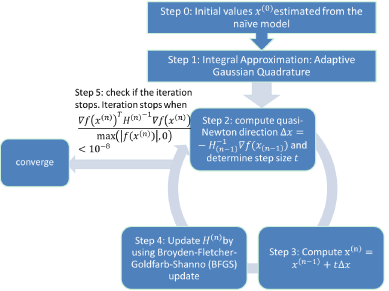Figure 1: Flow chart of computational algorithm to fit the integrated model based on adaptive Gaussian quadrature method and dual quasi-Newton algorithm. Step 0: estimate the initial value of Ø ,0,σ1, denoted as 𝑥(0), by using the naïve model; Step 1: generate the approximate likelihood function by using adaptive Gaussian Quadrature method; Step 2: compute the quasi-Newton direction Δx, determine the step size 𝑡 to satisfy the Goldstein conditions; Step 3: update parameters 𝑥 value; Step 4: update Hessian matrix; Step 5: check if the iteration stops. If not, go to Step 2, if yes, the iteration stops. A grid searching with center from the estimates of naïve model was applied in our algorithm.